# MCAT Physical : Bernoulli's Equation

## Example Questions

### Example Question #1 : Bernoulli's Equation

A liquid of density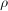enters a pipe at velocity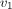and with pressure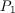. The liquid then exits the pipe a height h above the starting point, at velocity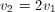. What is the pressure,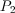, at this exit point, in terms of,, and h?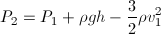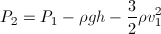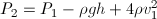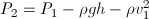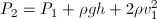Explanation:

Use Bernoulli's equation: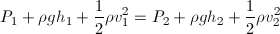Plug in our given values: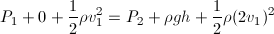Rearrange to isolate### Example Question #11 : Flow

Which of the following is the gravitational equivalent in mechanical energy to Bernoulli's equation for fluid mechanics?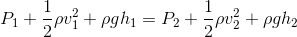Potential energy

Electrostatic energy

Kinetic energy

Gauge pressure

Internal energy

Potential energy

Explanation:

Bernoulli's equation states that:Essentially, this equation notes that pressure, velocity, and height of a fluid during flow can be related by a constant term. Very similarly, kinetic energy and potential energy sum to a constant mechanical energy for static compounds.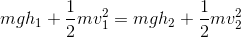Neglecting the term for pressure in Bernoulli's equation, there are direct correlations between kinematic and gravitational terms. Each term has an equivalent in both equations.

Kinematic terms: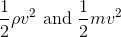Gravitational terms: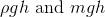In Bernoulli's equation, kinetic energy is the kinematic equivalent and potential energy is the gravitational equivalent.

### Example Question #3 : Bernoulli's Equation

Which of the following is/are assumptions of the Bernoulli equation?

I. The fluid is flowing turbulently

II. The volume of the fluid changes when pressure is applied

III. The fluid is frictionless

I only

III only

I and III

I, II, and III

III only

Explanation:

Bernoulli’s principle is an equation that states the relationship between the pressure and velocity of a fluid flowing through a pipe.It operates on three main assumptions. First, the fluid has to have laminar flow. There are two types of flow: laminar and turbulent. Laminar flow is characterized by uniform and ordered flow, whereas turbulent flow is characterized by haphazard and irregular flow. For the Bernoulli equation, the fluid must flow uniformly (laminar). Second, the fluid must be incompressible. This means that the fluid must not change volume (should not be compressed) when an outside pressure is applied. Third, the fluid must experience no friction during flow. Recall that during fluid flow friction usually occurs between layers of fluid because the molecules from each layer interact with each other; however, for the Bernoulli equation to be valid this friction between layers of fluid must be negligible (must be frictionless).

### Example Question #4 : Bernoulli's Equation

A researcher is observing a fluid flowing in a pipe. He measures the velocity of the flow at both ends of the pipe and wants to know the relative pressures each end. Which of the following equations will be most useful to him?

Doppler effect

Bernoulli’s equation

Continuity equation

Poiseuille’s law

Bernoulli’s equation

Explanation:

The question states that the researcher wants to know the relationship between velocity and pressure. Recall that the Bernoulli equation states this relationship for a fluid flowing through a pipe; therefore, the most appropriate principle for the researcher would be Bernoulli’s equation.

Poiseuille’s law and the continuity equation are both fluid mechanics principles, but neither of them state a relationship between velocity and pressure. Poiseuille’s law states the relationship between flow resistance and flow rate for a fluid flowing through a pipe, whereas the continuity equation states the relationship between velocity of the fluid and the area of the pipe. The Doppler effect is irrelevant to this question because it relates changes in frequency of a wave relative to the observer and the wave source; it is not a fluid mechanics principle.

Bernoulli equation:Poiseulle's law: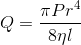Continuity equation: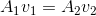Doppler effect: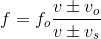### Example Question #5 : Bernoulli's Equation

A fluid is flowing through a pipe from left to right. If you increase the vertical height of the left end, what will happen to the pressure and velocity of the fluid at the left end?

Bernoulli’s equation:Pressure will increase but velocity will decrease

Pressure and velocity will remain constant

The relative changes to pressure and velocity cannot be determined from the given information

Pressure and velocity will decrease

The relative changes to pressure and velocity cannot be determined from the given information

Explanation:

To answer this question you need to know the Bernoulli equation:In the equation,is pressure,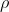is density,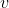is velocity,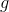is acceleration due to gravity, andis vertical height. Let’s assume that the left side of this equation is for the left end of the pipe and right side is for the right end of the pipe.

The question states that the vertical height,, is increased at the left end; therefore, the potential energy term on the left hand side of Bernoulli’s equation is increased (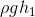). Since the potential energy at the left end increased, the other term(s) on the left hand side of the equation must decrease so that the left hand side equals the right hand side.

This means that the sum of pressure and velocity must decrease; however, we do not have enough information to determine the kinds of changes that will occur in each individual term. It is possible that only one term decreases, while the other term stays constant, or it is possible that both terms decrease; therefore, without more information, we cannot determine the relative changes to pressure and velocity.

### Example Question #6 : Bernoulli's Equation

Due to plaque buildup, a small part of a patient’s aorta has a smaller radius than a regular, healthy aorta. Which of the following is true regarding the unhealthy and healthy aorta?

Bernoulli's equation:Continuity equation:Flow in the clogged part of the unhealthy aorta will have a higher velocity and lower pressure than in a healthy aorta

Flow in the clogged part of the unhealthy aorta will have a lower velocity and higher pressure than in a healthy aorta

Flow in the clogged part of the unhealthy aorta will have a lower velocity and lower pressure than in a healthy aorta

Flow in the clogged part of the unhealthy aorta will have a higher velocity and higher pressure than in a healthy aorta

Flow in the clogged part of the unhealthy aorta will have a higher velocity and lower pressure than in a healthy aorta

Explanation:

The question states that a part of the unhealthy aorta has a smaller radius. This means that this portion of the aorta will have a smaller cross-sectional area. To solve this question we need to use both the continuity equation and the Bernoulli equation. The continuity equation is as follows:andare area and velocity of fluid flow, respectively. This equation states that the product of area and velocity of fluid flow on one side of a pipe must equal the product of area and velocity of the fluid flow on the other side of the pipe. Since part of the unhealthy aorta has a smaller radius, it will have a smaller area. According to the continuity equation, the velocity of fluid flowing through the smaller part of the aorta will increase to compensate for the decrease in area; therefore, the velocity of the fluid flow will increase in the clogged region of the aorta.

The second equation we need to use is the Bernoulli equation:Here,is pressure,is density,is velocity,is acceleration due to gravity, andis vertical height. Bernoulli’s equation states that an increase in velocity of fluid flow will decrease the pressure. This occurs because the pressure will decrease to compensate for the increase in velocity (to ensure that the left hand side of the equation equals the right hand side); therefore, the clogged region of the aorta will have a higher velocity and lower pressure.